## Example Questions

### Example Question #171 : Plane Geometry

The base of a triangle is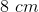and the area is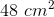.  The height of the triangle is then decreased by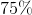. What is the final area of the triangle?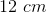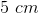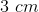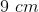Explanation:

The formula for the area of a triangle is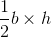.

If the area is equal to 48 cm2 and the base is 8 cm, then the initial height is: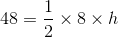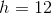If 12 is decreased by 75% then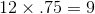, and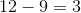. The final height is 3 cm.

Therefore the final area is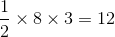.